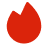# Goldman Sachs计算机科学面试真题

BQ

Coding（0）
System Design（0）
Algorithm（2）
Ood（0）高频题（0）

Coding（0）
System Design（0）
Algorithm（2）
Ood（0）高频题（0）
1.LeetCode 141
2.Array Game
3.LeetCode 161
1. LeetCode 141
Given head, the head of a linked list, determine if the linked list has a cycle in it.

There is a cycle in a linked list if there is some node in the list that can be reached again by continuously following the next pointer. Internally, pos is used to denote the index of the node that tail's next pointer is connected to. Note that pos is not passed as a parameter.

Return true if there is a cycle in the linked list. Otherwise, return false.

Example 1:

```Input: head = [3,2,0,-4], pos = 1
Output: true
Explanation: There is a cycle in the linked list, where the tail connects to the 1st node (0-indexed).
```
Example 2:

```Input: head = [1,2], pos = 0
Output: true
Explanation: There is a cycle in the linked list, where the tail connects to the 0th node.
```
Example 3:

```Input: head = , pos = -1
Output: false
Explanation: There is no cycle in the linked list.
```

Constraints:

• The number of the nodes in the list is in the range [0, 104].
• -105 <= Node.val <= 105
• pos is -1 or a valid index in the linked-list.

Follow up: Can you solve it using O(1) (i.e. constant) memory?

2. Array Game

3. LeetCode 161
Given two strings s and t, return true if they are both one edit distance apart, otherwise return false.

A string s is said to be one distance apart from a string t if you can:

Insert exactly one character into s to get t.

Delete exactly one character from s to get t.

Replace exactly one character of s with a different character to get t.

Example 1:

Input: s = "ab", t = "acb" Output: true Explanation: We can insert 'c' into s to get t.

Example 2:

Input: s = "", t = "" Output: false Explanation: We cannot get t from s by only one step.

Constraints:

0 <= s.length, t.length <= 104

s and t consist of lowercase letters, uppercase letters, and digits.